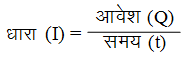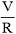Ex﻿

# 1. Electricity

#### Points to Be Remember (Electricity)

Points to Be Remember
Current : The rate of flow of charge (Q) through a conductor is called current. Current (I) is given by,
Current =or I =The SI unit of current is ampere (A) : 1A = 1 C/s
The current flowing through a circuit is measured by a device called ammeter. Ammeter is connected in series with the conductor. The direction of the current is taken as the direction of the flow of positive charge.
Ohm’s Law : At any constant temperature, the current (I) flowing through a conductor is directly proportional to the potential (V) applied across it.
Mathematically,
I =or V = IR
Resistance : Resistance is the property of a conductor by virtue of which it opposes the flow of electricity through it. Resistance is measured in ohms. Resistance is a scalar quantity.
Resistivity : The resistance offered by a cube of a substance having side of 1 meter, when current flows perpendicular to the opposite faces, is called its resistivity (ρ). The SI unit of resistivity is ohm.m. Equivalent Resistance : A single resistance which can replace a combination of resistances so that current through the circuit remains the same is called equivalent resistance.
Law of combination of resistances in series When a number of resistance are connected in series, their equivalent resistance is equal to the sum of the individual resistances.
If R1, R2, R3, etc. are combined in series, then the equivalent resistance (R) is given by,
R = R1 + R2 + R3 + .....
The equivalent resistance of a number of resistances connected in series is higher than each individual resistance.
Law of combination of resistances in parallel : When a number of resistances are connected in parallel, the reciprocal of the equivalent resistance is equal to the sum of the reciprocals of the individual resistances.
If R1, R2, R3, etc. are combined in parallel, then the equivalent resistance (R) is given by.The equivalent resistance of a number of resistances connected in parallel is less than each of all the individual resistances.

If you want to give information about online courses to other students, then share it with more and more on Facebook, Twitter, Google Plus. The more the shares will be, the more students will benefit. The share buttons are given below for your convenience.
×

#### NTSE Physics (Class X)

• Electricity
• Magnetic Effect of Curent
• Light Reflection
• Light Refraction
• Human Eye & Colourful World
• Source of Energy
• Motion

#### NTSE Physics (Class IX)

• Force & laws of motion
• Work and Energy
• Gravitation
• Sound

SHOW CHAPTERS